# Subtracting Geometric Vectors

Subtracting vectors results in a new Resultant Vector. Geometric and algebraic vectors can be subtracted using different techniques.

## Subtracting Geometric Vectors

Geometric vectors can be subtracted by positioning the vectors head to tail or tail to tail. Remember, you can move a vector around in space as long as it maintains its magnitude and direction. Subtracting vectors is the same as adding the Opposite vector.

## Subtracting Geometric Vectors Head to Tail

To subtract vectors head to tail, add the Opposite vector. Move the Opposite vector so that it's tail starts at the head of the positive vector. The Resultant Vector starts at the tail of the first vector and ends at the head of the last one.

Below are the steps to subtract vectors $$\vec{x}$$ and $$\vec{w}$$ head to tail. This method is referred to the Parallelogram Law of Vector Addition but we are adding the Opposite vector.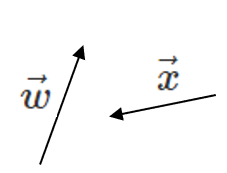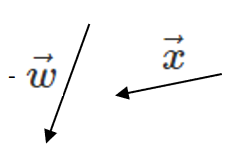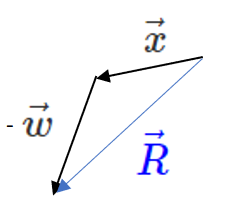The figure below shows how to add and subtract $$\vec{x}$$ and $$\vec{w}$$ :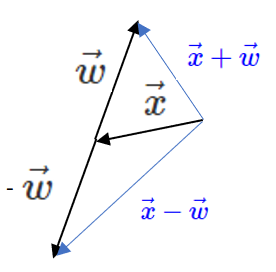Subtracting geometric vectors involves solving triangles using trigonometry equations. Below is a summary:

 Right Triangles Pythagorean Theorem $$c^2 = a^2 + b^2$$ SOH CAH TOA $$sin(\theta) = \frac{opp}{hyp} = \frac{y}{r}$$ $$cos(\theta) = \frac{adj}{hyp} = \frac{x}{r}$$ $$tan(\theta) = \frac{opp}{adj} = \frac{y}{x}$$
 Other Triangles Cosine Law $$c^2 = a^2 + b^2 - 2 a b cos(\theta)$$ Sin Law $$\frac{sin(A)}{a} = \frac{sin(B)}{b} = \frac{sin(C)}{c}$$
##### Try these questions:

A plane travels $$200 [\frac{km}{hr}] N 20^\circ E$$ when the wind speed is $$90 [\frac{km}{hr}] E$$. What is the plane's velocity when there is no wind?

## Subtracting Geometric Vectors Tail to Tail

To subtract vectors tail to tail, move the negative vector so that it's tail starts at the tail of the positive vector. The Resultant Vector starts at the head of the negative vector and ends at the head of the positive vector.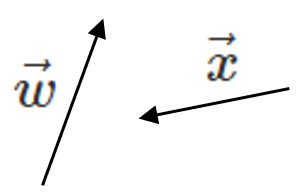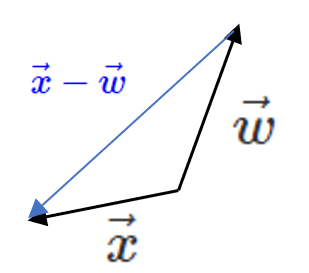The figure below shows how to add and subtract $$\vec{x}$$ and $$\vec{w}$$ :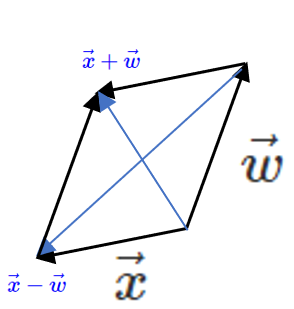In parallelogram ABCDE, what is $$\vec{AB} - \vec{AD}$$?